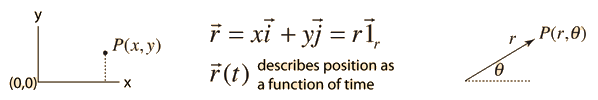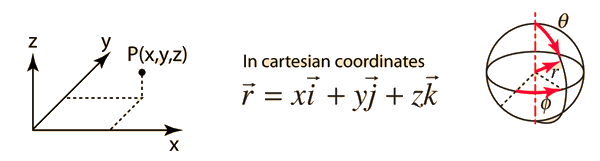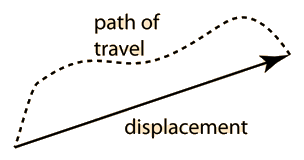# Position

Specifying the position of an object is essential in describing motion. In one dimension some typical ways arex(t) is used to represent position as a function of time.
In two dimensions, either cartesian or polar coordinates may be used, and the use of unit vectors is common. A position vector r may be expressed in terms of the unit vectors.In three dimensions, cartesian or spherical polar coordinates are used, as well as other coordinate systems for specific geometries.The vector change in position associated with a motion is called the displacement.

Index

 HyperPhysics***** Mechanics R Nave
Go Back

# Displacement

The displacement of an object is defined as the vector distance from some initial point to a final point. It is therefore distinctly different from the distance traveled except in the case of straight line motion in one direction. The distance traveled divided by the time is called the speed, while the displacement divided by the time defines the average velocity.If the positions of the initial and final points are known, then the distance relationship can be used to find the displacement.
Index

 HyperPhysics***** Mechanics R Nave
Go Back